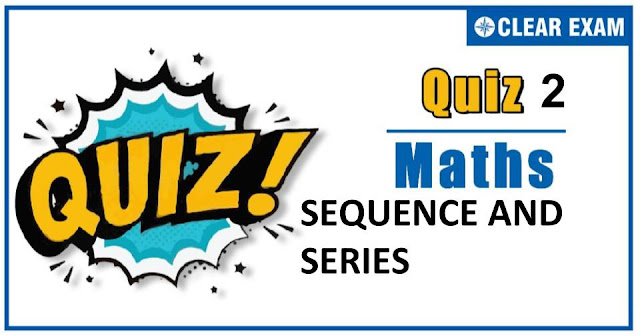## [LATEST]\$type=sticky\$show=home\$rm=0\$va=0\$count=4\$va=0

As per analysis for previous years, it has been observed that students preparing for JEE MAINS find Mathematics out of all the sections to be complex to handle and the majority of them are not able to comprehend the reason behind it. This problem arises especially because these aspirants appearing for the examination are more inclined to have a keen interest in Mathematics due to their ENGINEERING background.

Furthermore, sections such as Mathematics are dominantly based on theories, laws, numerical in comparison to a section of Engineering which is more of fact-based, Physics, and includes substantial explanations. By using the table given below, you easily and directly access to the topics and respective links of MCQs. Moreover, to make learning smooth and efficient, all the questions come with their supportive solutions to make utilization of time even more productive. Students will be covered for all their studies as the topics are available from basics to even the most advanced.

Q1. The sum of the series 1^2-2^3+3^2-4^2+5^2-6^2+⋯-2008^2+2009^2 is
•  2019045
•  1005004
•  2000506
•  None of these
Solution

Q2.The sum to n terms of the series 1+3/2+7/4+15/8+31/16+⋯, is
•  2(n-1)+1/(2n-1)
•  2n-1/2^n
•  2+1/2^n
•  2n-1+1/2^n
Solution
Let S_n=1+3/2+7/4+15/8+31/16+⋯to n terms ⇒S_n=1+(2^2-1)/2+(2^3-1)/2^2 +(2^4-1)/2^3 +(2^5-1)/2^4 +⋯+(2^n-1)/2^(n-1) ⇒S_n=(2-1)+(2-1/2)+(2-1/2^2 )+(2-1/2^3 )+(2-1/2^4 )+⋯+(2-1/2^(n-1) ) ⇒S_n=2n-(1+1/2+1/2^2 +⋯1/2^(n-1) ) ⇒S_n=2n-((1-1/2^n )/(1-1/2))=2(n-1)+1/2^(n-1)

Q3.  The first two terms of a geometric progression add upto 12. The sum of the third and the fourth terms is 48. If terms of the geometric progression are alternately positive and negative, then the first term is
•   4
•  -4
•  -12
•  12
Solution
Since, a+ar=a(1+r)=12 …(i) and ar^2+ar^3=ar^2 (1+r)=48 …(ii) From Eqs. (i) and (ii), r^2=4 ⇒ r=-2 (Since, the series is alternately sign, so we take negative values). On putting the value of r in Eq. (i), we get a=-12

Q4. If, for 0
•  0
•  2+√3
•  2-√3
•  None of these
Solution
For 0

Q5.1/2.5+1/5.8+1/8.11+...upto n terms is equal to
•  n/(4n+6)
•  1/(6n+4)
•  n/(6n+4)
•  n/(3n+7)
Solution
Let S_n=1/2.5+1/5.8+1/8.11+...+1/((3n-1)(3n+2)) =1/3 [1/2-1/5+1/5-1/8+.…..+1/(3n-1)-1/(3n+2)] =1/3 [1/2-1/(3n+2)]=n/(6n+4)

Q6. If e^x/(1-x)=B_0+B_1 x+B_2 x^2+⋯+B_n x^n+⋯, then B_n-B_(n-1) equals
•  1/(n !)
•  1/((n-1) !)
•   1/(n !)-1/((n-1) !)
•  1
Solution
We have, e^x/(1-x)=B_0+B_1 x+B_2 x^2+⋯+B_n x^n+⋯ ⇒∑_(r=0)^∞▒x^r/(r !)=(B_0+B_1 x+B_2 x^2+⋯+B_n x^n+⋯)(1-x) On equating the coefficients of x^n on both sides, we get 1/(n !)=B_n-B_(n-1)

Q7.The sum of the infinite series 1/2 (1/3+1/4)-1/4 (1/3^2 +1/4^2 )+1/6 (1/3^3 +1/4^3 )- … is equal to
•  a) 1/2 log⁡2
•  log⁡〖3/5〗
•  log⁡〖5/3〗
•  1/2 log⁡〖5/3〗
Solution
(d) 1/2 (1/3+1/4)-1/4 (1/3^2 +1/4^2 )+1/6 (1/3^3 +1/4^3 )-… =1/2 [1/3-1/2 (1/3^2 )+1/3 (1/3^3 )-...]+1/2 [1/4-1/2 (1/4^2 )+1/3 (1/4^3 )-...] =1/2 [log⁡(1+1/3) ]+1/2 [log⁡(1+1/4) ] =1/2 [log⁡〖(4/3)×(5/4)〗 ] =1/2 log⁡(5/3)

Q8.1/2-1/〖2.2〗^2 +1/〖3.2〗^3 -1/〖4.2〗^4 +⋯ is equal to
•  1/4
•  log_3⁡(3/4)
•  log_e⁡(3/2)
•  log_e⁡(2/3)
Solution
On putting x=1/2 in log_e⁡〖(1+x)〗=x-x^2/2+x^3/3-x^4/4+...∞ We get, 1/2-1/〖2.2〗^2 +1/〖3.2〗^3 -1/〖4.2〗^4 +...=log_e⁡(1+1/2)=log_e⁡(3/2)

Q9.If a,b,c are in A.P., b-a,c-b and a are in G.P., then a:b:c is
•  1:2:3
•  1:3:5
•  2:3:4
•  1:2:4
Solution
It is given that a,b,c are in A.P. and (b-a),(c-b),a are in G.P. ∴2b=a+c And, (c-b)^2=(b-a)a ⇒(b-a)^2=(b-a)a [2b=a+c⇒b-a=c-b] ⇒b=2a ⇒c=3 a [Using∶2b=a+c] ⇒a∶b∶c=1∶2∶3

Q10. If the first, second and last terms of an arithmetic series are a,b and c respectively, then the number of terms is
•  (b+c-2a)/(b-a)
•  (b+c+2a)/(b-a)
•   (b+c-2a)/(b+a)
•  (b+c+2a)/(b+a)
Solution
Since, l=A+(n-1)d ∴c=a+(n-1)(b-a) ⇒(n-1)=(c-a)/(b-a) ⇒n=(b+c-2a)/(b-a)#### Written by: AUTHORNAME

AUTHORDESCRIPTION## Want to know more

Please fill in the details below:

## Latest NEET Articles\$type=three\$c=3\$author=hide\$comment=hide\$rm=hide\$date=hide\$snippet=hide

Name

ltr
item
BEST NEET COACHING CENTER | BEST IIT JEE COACHING INSTITUTE | BEST NEET & IIT JEE COACHING: SEQUENCE AND SERIES QUIZ-2
SEQUENCE AND SERIES QUIZ-2
https://1.bp.blogspot.com/-leAGu0OzvbA/X7EHc62PxYI/AAAAAAAAN0g/IB2r9gJeXjkhq-hg3uGNWBIaImr8HtY-QCLcBGAsYHQ/w640-h336/Quiz%2BImage%2B20%2B%25288%2529.jpg
https://1.bp.blogspot.com/-leAGu0OzvbA/X7EHc62PxYI/AAAAAAAAN0g/IB2r9gJeXjkhq-hg3uGNWBIaImr8HtY-QCLcBGAsYHQ/s72-w640-c-h336/Quiz%2BImage%2B20%2B%25288%2529.jpg
BEST NEET COACHING CENTER | BEST IIT JEE COACHING INSTITUTE | BEST NEET & IIT JEE COACHING
https://www.cleariitmedical.com/2020/11/sequence%20and%20series%20quiz-2.html
https://www.cleariitmedical.com/
https://www.cleariitmedical.com/
https://www.cleariitmedical.com/2020/11/sequence%20and%20series%20quiz-2.html
true
7783647550433378923
UTF-8

STAY CONNECTED